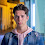Rotate an array of n elements to the right by k steps. For example, with n = 7 and k = 3, the array [1,2,3,4,5,6,7] is rotated to [5,6,7,1,2,3,4]. How many different ways do you know to solve this problem?

### Using intermediate array

1. First find out correct number of rotations using mod
2. Create temporary array
3. Push array elements into that temporary array
4. Copy that temporary array into  original array
```function rotateArray(nums,k) {
if(isNaN(k) || k < 0) {
throw "provide valid order";
}

if(nums.length == 0) {
throw "provide valid array";
}

if(k > nums.length)
k=k%nums.length;

var result = [];

for(var i=0; i < k; i++){
result[i] = nums[nums.length-k+i];
}

var j=0;
for(var i=k; i<nums.length; i++){
result[i] = nums[j];
j++;
}

nums = result.slice();
console.log(nums);
}
```

#### Complexity

Observe above demo. It will take O(n) space and O(n) time

### Using bubble rotate

By using bubble swap, we can solve this problem. Move array elements from right side to left side of the array using bubble swap
```function rotateArray2(arr, order) {
if(isNaN(order) || order < 0) {
throw "provide valid order";
}

if(arr.length == 0) {
throw "provide valid array";
}
for (var i = 0; i < order; i++) {
for (var j = arr.length - 1; j > 0; j--) {
var temp = arr[j];
arr[j] = arr[j - 1];
arr[j - 1] = temp;
}
}
console.log(arr);
}```

#### Complexity

Observe above demo. It will take O(1) space and O(n*k) time

### Using Reversal

Divide array into 2 parts based on given order. Reverse first part and then reverse second, then reverse whole array
```function rotate3(arr, order) {
if(isNaN(order) || order < 0) {
throw "provide valid order";
}

if(nums.length == 0) {
throw "provide valid array";
}

order = order % arr.length;

//length of first part
var a = arr.length - order;

reverse(arr, 0, a-1);
reverse(arr, a, arr.length-1);
reverse(arr, 0, arr.length-1);
console.log(arr);
}

function reverse(arr, left, right){
while(left < right){
var temp = arr[left];
arr[left] = arr[right];
arr[right] = temp;
left++;
right--;
}
}

rotate3([1,2,3,4,5,6],3);
```

#### Complexity

Observe above demo. It will take O(1) space and O(n) time

1.This comment has been removed by the author.

2.3.Custom Organometal Halide Perovskite Quantum Dots - We offer a bright and efficient portfolio for printed electronics, leading the industry in nanoparticle engineering and formulation. We can help you develop and commercialize new product designs. Time-to-market is minimized with rapid material screening and robust quality control processes. This ensures that only the highest quality materials are released to the electronics markets.

## BlogrollSrinivas Dasari find me on facebook follow me on twitter